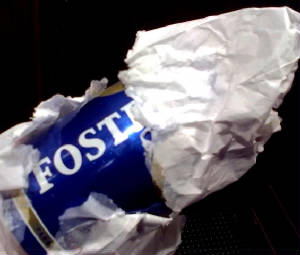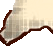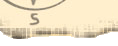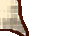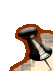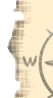WELCOMEINDEXCOOKIESCAKES, mixes & layersCHOCOLATEcreams mousses puddingsDOUGHSIce Creams Sorbets ..Sauces SyrupsSAVORIESDRINKS

 BEVERAGESBAR BASICS ___on this page___ LIQUORS & Hard StuffVARIETALSWORD

A  B  C  D  E  F  G  H  I  J  K  L  M  N  O  P  Q  R  T  U  V  W  X  Y  Z
Absinthe -

A  B  C  D  E  F  G  H  I  J  K  L  M  N  O  P  Q  R  T  U  V  W  X  Y  Z

A  B  C  D  E  F  G  H  I  J  K  L  M  N  O  P  Q  R  T  U  V  W  X  Y  Z

A  B  C  D  E  F  G  H  I  J  K  L  M  N  O  P  Q  R  T  U  V  W  X  Y  Z

A  B  C  D  E  F  G  H  I  J  K  L  M  N  O  P  Q  R  T  U  V  W  X  Y  Z

A  B  C  D  E  F  G  H  I  J  K  L  M  N  O  P  Q  R  T  U  V  W  X  Y  Z

A  B  C  D  E  F  G  H  I  J  K  L  M  N  O  P  Q  R  T  U  V  W  X  Y  Z

A  B  C  D  E  F  G  H  I  J  K  L  M  N  O  P  Q  R  T  U  V  W  X  Y  Z

A  B  C  D  E  F  G  H  I  J  K  L  M  N  O  P  Q  R  T  U  V  W  X  Y  Z

A  B  C  D  E  F  G  H  I  J  K  L  M  N  O  P  Q  R  T  U  V  W  X  Y  Z

A  B  C  D  E  F  G  H  I  J  K  L  M  N  O  P  Q  R  T  U  V  W  X  Y  Z

A  B  C  D  E  F  G  H  I  J  K  L  M  N  O  P  Q  R  T  U  V  W  X  Y  Z

A  B  C  D  E  F  G  H  I  J  K  L  M  N  O  P  Q  R  T  U  V  W  X  Y  Z

A  B  C  D  E  F  G  H  I  J  K  L  M  N  O  P  Q  R  T  U  V  W  X  Y  Z

A  B  C  D  E  F  G  H  I  J  K  L  M  N  O  P  Q  R  T  U  V  W  X  Y  Z

A  B  C  D  E  F  G  H  I  J  K  L  M  N  O  P  Q  R  T  U  V  W  X  Y  Z

A  B  C  D  E  F  G  H  I  J  K  L  M  N  O  P  Q  R  T  U  V  W  X  Y  Z

OUPS  !

A  B  C  D  E  F  G  H  I  J  K  L  M  N  O  P  Q  R  T  U  V  W  X  Y  Z

A  B  C  D  E  F  G  H  I  J  K  L  M  N  O  P  Q  R  S  T  U  V  W  X  Y  Z

A  B  C  D  E  F  G  H  I  J  K  L  M  N  O  P  Q  R  T  U  V  W  X  Y  Z

A  B  C  D  E  F  G  H  I  J  K  L  M  N  O  P  Q  R  T  U  V  W  X  Y  Z

OUPS  !

A  B  C  D  E  F  G  H  I  J  K  L  M  N  O  P  Q  R  T  U  V  W  X  Y  Z

A  B  C  D  E  F  G  H  I  J  K  L  M  N  O  P  Q  R  T  U  V  W  X  Y  Z

A  B  C  D  E  F  G  H  I  J  K  L  M  N  O  P  Q  R  T  U  V  W  X  Y  Z

- -

A  B  C  D  E  F  G  H  I  J  K  L  M  N  O  P  Q  R  T  U  V  W  X  Y  Z

A  B  C  D  E  F  G  H  I  J  K  L  M  N  O  P  Q  R  T  U  V  W  X  Y  Z

- Zinfandel - -

______________________________________________________________________back to top ▲TROMPE L'OEIL

- Egg_Nog

- Hot ChocolateHISTORY OF SPIRITS

- Brandy

- Liquor

- Port

- Rum

- Tequila

- Vodka

- Whiskey